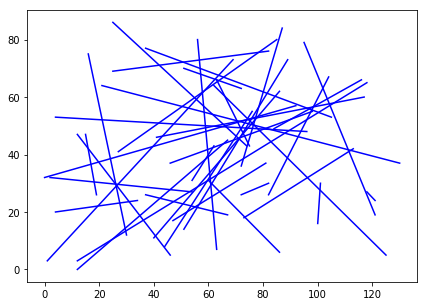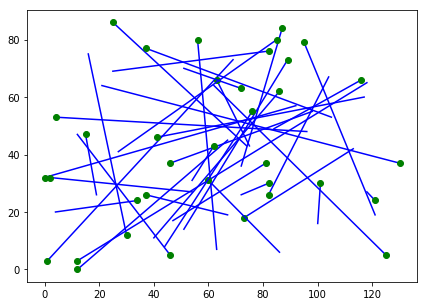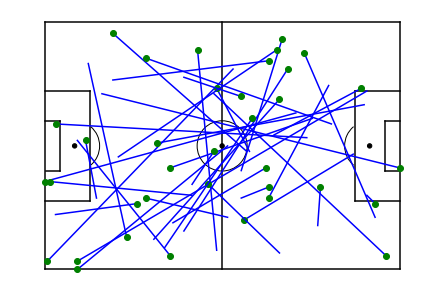# Drawing a Pass Map in Python

Pass maps are an established visualisation in football analysis, used to show the area of the pitch where a player made their passes. You’ll find examples across the Football Manager series, TV coverage, and pretty much all formats of football journalism. Similar plots are used to show shots or other events in a game, and multiple other sports make use of similar maps of what goes on during a game. This article runs through one way to create these in Python, making use of the Matplotlib library. Let’s fire up our modules, open our dataset and take a look at what we are working with:

In :
```import pandas as pd
import numpy as np
import matplotlib.pyplot as plt
from matplotlib.patches import Arc

%matplotlib inline

```
Out:
Half Time Event Player Team Xstart Ystart Xend Yend
0 First Half 67.0 Pass Zeedayne France 12 3 118 65
1 First Half 70.2 Pass Zeedayne France 82 30 72 26
2 First Half 78.5 Pass Zeedayne France 1 3 69 73
3 First Half 106.5 Pass Zeedayne France 41 46 117 60
4 First Half 115.7 Pass Zeedayne France 34 24 4 20

*** Plotting Lines

Our dataset contains Zeedayne’s passes from her match. We have when they happened, in additon to the starting and ending X and Y locations. With this information, matplotlib makes it easy to draw lines. We can use the ‘.plot()’ function to draw lines if we give it two lists:

• List one must contain the start and end X locations
• List two gives the start and end Y locations

For example, plt.plot([0,1],[2,3] will plot a line from location (0,2) to (1,3).

We could write this line to plot each of Zeedayne’s passes, but we hate repeating ourselves and are a little bit lazy, so let’s use a for loop to do this. Take a look at our code below to see it in action:

In :
```fig, ax = plt.subplots()
fig.set_size_inches(7, 5)

for i in range(len(data)):
plt.plot([int(data["Xstart"][i]),int(data["Xend"][i])],
[int(data["Ystart"][i]),int(data["Yend"][i])],
color="blue")

plt.show()
```Great job on plotting all of the passes! Unfortunately, we do not know where they happened on the pitch, or the direction, or much else, but we will get there!

Let’s start with adding a circle at the starting point of each pass to understand the direction. This is as easy as before, we just plot the start data, like below:

In :
```fig, ax = plt.subplots()
fig.set_size_inches(7, 5)

for i in range(len(data)):
plt.plot([int(data["Xstart"][i]),int(data["Xend"][i])],
[int(data["Ystart"][i]),int(data["Yend"][i])],
color="blue")

plt.plot(int(data["Xstart"][i]),int(data["Ystart"][i]),"o", color="green")

plt.show()
```Another massive and easy improvement would be to add a pitch map – as our article here explains. Let’s steal the code and add the pitch here – obviously feel free to steal the pitch too!

In :
```#Create figure
fig=plt.figure()
fig.set_size_inches(7, 5)

#Pitch Outline & Centre Line
plt.plot([0,0],[0,90], color="black")
plt.plot([0,130],[90,90], color="black")
plt.plot([130,130],[90,0], color="black")
plt.plot([130,0],[0,0], color="black")
plt.plot([65,65],[0,90], color="black")

#Left Penalty Area
plt.plot([16.5,16.5],[65,25],color="black")
plt.plot([0,16.5],[65,65],color="black")
plt.plot([16.5,0],[25,25],color="black")

#Right Penalty Area
plt.plot([130,113.5],[65,65],color="black")
plt.plot([113.5,113.5],[65,25],color="black")
plt.plot([113.5,130],[25,25],color="black")

#Left 6-yard Box
plt.plot([0,5.5],[54,54],color="black")
plt.plot([5.5,5.5],[54,36],color="black")
plt.plot([5.5,0.5],[36,36],color="black")

#Right 6-yard Box
plt.plot([130,124.5],[54,54],color="black")
plt.plot([124.5,124.5],[54,36],color="black")
plt.plot([124.5,130],[36,36],color="black")

#Prepare Circles
centreCircle = plt.Circle((65,45),9.15,color="black",fill=False)
centreSpot = plt.Circle((65,45),0.8,color="black")
leftPenSpot = plt.Circle((11,45),0.8,color="black")
rightPenSpot = plt.Circle((119,45),0.8,color="black")

#Draw Circles

#Prepare Arcs
leftArc = Arc((11,45),height=18.3,width=18.3,angle=0,theta1=310,theta2=50,color="black")
rightArc = Arc((119,45),height=18.3,width=18.3,angle=0,theta1=130,theta2=230,color="black")

#Draw Arcs

#Tidy Axes
plt.axis('off')

for i in range(len(data)):
plt.plot([int(data["Xstart"][i]),int(data["Xend"][i])],[int(data["Ystart"][i]),int(data["Yend"][i])], color="blue")
plt.plot(int(data["Xstart"][i]),int(data["Ystart"][i]),"o", color="green")

#Display Pitch
plt.show()
```Awesome, now we can see Zeedayne’s pass locations – seems to cover just about everywhere!

### Summary

Plotting simple pass maps is pretty easy – we just need to use matplotlib’s ‘.plot’ functionality to draw our lines, and a for loop to run through X/Y origin and destiniation data to plot each line.

On their own, they do not offer much information, but once we add start location and a pitch map, we start to see where a player played their passes, where they ended up and the range that they employed in the match.

To develop on this, we can look to colour code our lines for success, or another variable. We could even look to plot a heatmap to show where a player was active. Watch out for a further article on these!